Next: Conservation of momentum Up: Conservation of energy Previous: Worked example 5.5: Sliding

## Worked example 5.6: Driving up an incline

Question: A car of weight 3000N possesses an engine whose maximum power output is 160kW. The maximum speed of this car on a level road is 35m/s. Assuming that the resistive force (due to a combination of friction and air resistance) remains constant, what is the car's maximum speed on an incline of 1 in 20 (i.e., ifis the angle of the incline with respect to the horizontal, then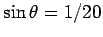)?

Answer: When the car is traveling on a level road at its maximum speed,, then all of the power output,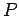, of its engine is used to overcome the power dissipated by the resistive force,. Hence,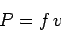where the left-hand side is the power output of the engine, and the right-hand side is the power dissipated by the resistive force (i.e., minus the rate at which this force does work on the car). It follows that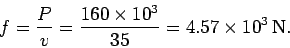When the car, whose weight is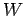, is traveling up an incline, whose angle with respect to the horizontal is, it is subject to the additional force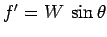, which acts to impede its motion. Of course, this force is just the component of the car's weight acting down the incline. Thus, the new power balance equation is written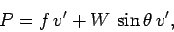where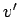is the maximum velocity of the car up the incline. Here, the left-hand side represents the power output of the car, whereas the right-hand side represents the sum of the power dissipated by the resistive force and the power expended to overcome the component of the car's weight acting down the incline. It follows that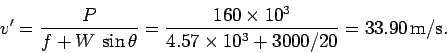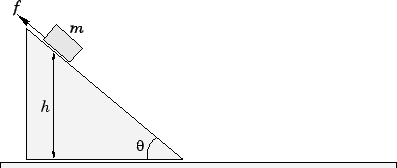Next: Conservation of momentum Up: Conservation of energy Previous: Worked example 5.5: Sliding
Richard Fitzpatrick 2006-02-02